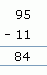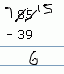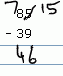Email us to get an instant 20% discount on highly effective K-12 Math & English kwizNET Programs!

#### Online Quiz (WorksheetABCD)

Questions Per Quiz = 2 4 6 8 10

### Grade 1 - Mathematics3.22 Subtraction - Double Digit (WIZ Math)

Subtraction without borrow
Method:
1. Start from the units place
2. Subtract the numbers in the units place
3. Subtract the numbers in the tens place

Example:
 Question Step 1 Step 2Subtraction with borrow
Method:
1. Look at the numbers in the units.
2. If the number on the bottom is more than the number at the top. Borrow 1 from the tens place.
3. Decrease 1 from the tens place and add 10 to units place.
4. Now perform subtraction for the units place.
5. Look at the numbers in the tens place
6. Perform similar subtraction for the tens place
Example:

 Question Step 1 Step 2The units digits, 5 is less than 9, therefore borrow 1 from tens place that is from 8.
Decrease 1 from 8 and add 10 to units place to become 15.
Subtract 15 and 9 to get 6.
Subtract 7 and 3 to get 4.

Directions: Answer the following questions. Also write at least ten examples for subtraction without borrow and subtraction with borrow of your own.
 Question 1: 57 - 14

Question 2: This question is available to subscribers only!

Question 3: This question is available to subscribers only!

Question 4: This question is available to subscribers only!

Question 5: This question is available to subscribers only!

Question 6: This question is available to subscribers only!